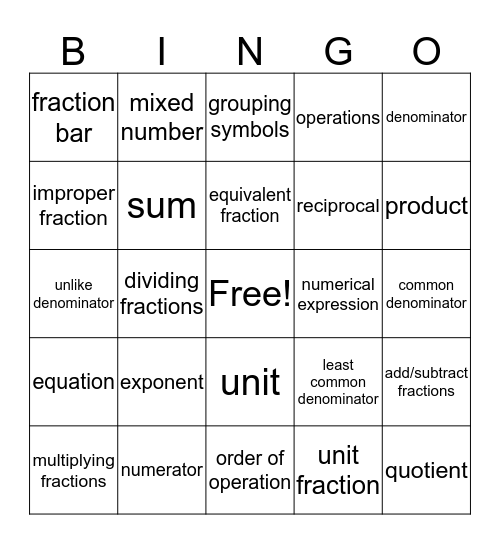# Vocab Final 3 BingoThis bingo card has a free space and 26 words: unit, unit fraction, equivalent fraction, numerator, denominator, fraction bar, improper fraction, mixed number, common denominator, least common denominator, reciprocal, unlike denominator, add/subtract fractions, multiplying fractions, dividing fractions, simplify, operations, equation, grouping symbols, quotient, product, order of operation, numerical expression, exponent, sum and difference.

## Play Online

Share this URL with your players:For more control of your online game, create a clone of this card first.

## Probabilities

With players vying for a you'll have to call about __ items before someone wins. There's a __% chance that a lucky player would win after calling __ items.

Tip: If you want your game to last longer (on average), add more unique words/images to it.